1603198800

# Time Complexity and Big O

## Introduction

Time should always be on a programmer’s mind. Namely, saving users and customers more of it. The less time users spend waiting, the more time they spend doing useful things with your product or service.

Just as with all aspects of life, there are many ways to solve a programming challenge. And — just as with real life — the method you choose could directly affect how long it takes to solve your problem. In this article, we’re going to explore the concept of efficiency within computer science and learn some ways to measure and describe this efficiency. By understanding how efficient our algorithms are, we can get a sense of where we may want to refactor our processes in order to get faster runtimes.

## The Concepts

If you’re already familiar with the concepts of time complexity and Big O and just need a quick refresher on how to calculate them, skip ahead to the “Finding Complexity” section.

Okay, so we need a way to easily compare two algorithms in terms of their efficiency. We could try comparing the actual runtimes of our functions but this method has problems. We can’t directly and consistently answer the question “how much time does it take for my code to run?” because it depends on too many variables. How fast your computer is, whether you’re running other applications at the same time, what programming language you’re using, are all factors in determining the actual runtime of your code. So instead of asking this question directly, we ask a similar question: “how does the runtime of my function grow as the size of my input grows?”. This turns out to be a much more important question to answer when thinking about how our algorithm will perform at scale, as opposed to comparing a single runtime of one algorithm to a single runtime of another which really isn’t giving us all the information we need. For example, we could have an algorithm that has a very fast runtime for small inputs, but that time could increase exponentially as the size of our input increases.

### Time Complexity

The term we use to describe how our algorithm performs under varying input sizes is its time complexity. Time complexity is a way of labeling — in plain English — how the runtime of a function increases as the size of our input increases. Some examples of time complexity labels are constant time, logarithmic time, linear time, and quadratic time (just to name a few). These labels describe the relationship between the size of our input and our runtime, and you might notice that they also describe the shape of various types of lines on a two-dimensional plot. This gives us a great, easy way to visualize how our runtime changes in regards to the input size. More on this in a bit.

### Big O

Big O notation is a way of describing this relationship mathematically, and it looks like this: O(1), O(log n), O(n), O(n²), etc., all representing a different time complexity relationship (constant time, logarithmic time, etc.).

We can use Big O notation to describe time complexity under best, average, and worst-case scenarios, but we’re almost always only concerned with the worst case. Like we’ve said, an algorithm can have very fast runtimes for small inputs (best case), but drastically worse runtimes for larger ones (worst case) so it’s always important to know how your code is going to perform under the most trying circumstances.

Quick note: It’s extremely common to be asked to analyze the complexity of your algorithm in technical/coding interviews. They are typically expecting an answer in Big O notation.

#programming #computer-science #data-science #big-o-notation

## Buddha Community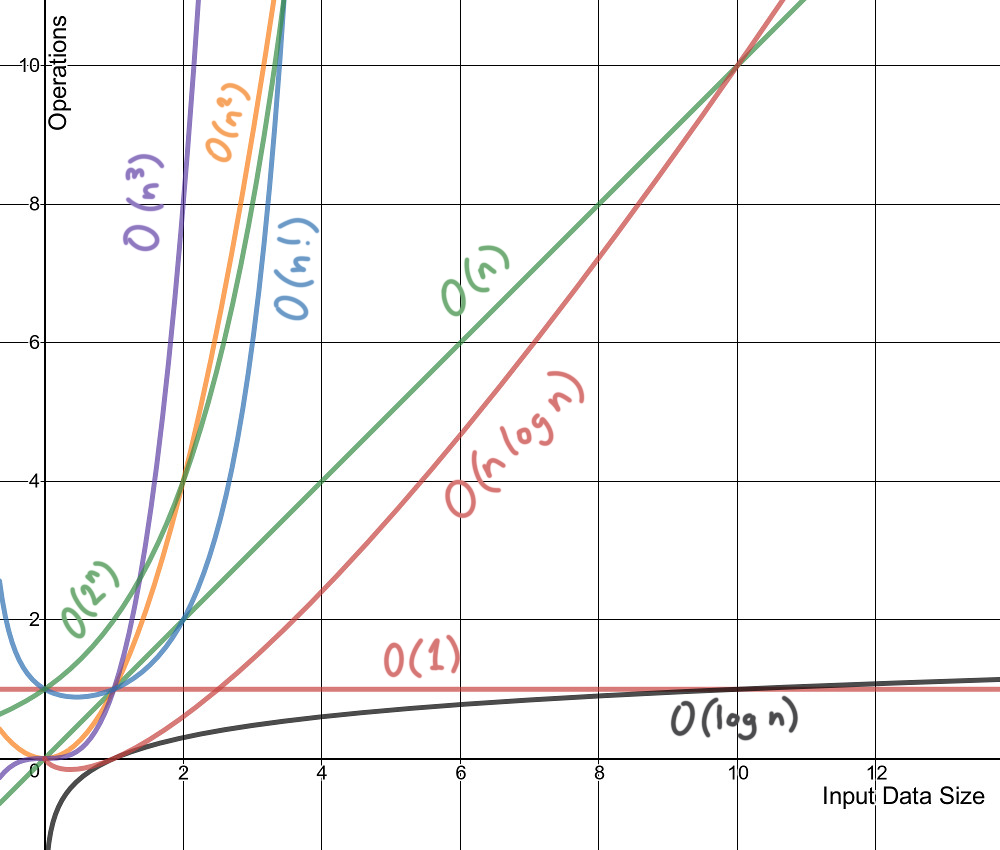1625640780

## Time Complexity & Big O notation | DSA-One Course - All you Need in One place

Hey guys, In this video, we’ll be talking about Time complexity and Big O notation. This is the first video of our DSA-One Course. We’ll also learn how to find the time complexity of Recursive problems.

Instagram: https://www.instagram.com/Anuj.Kumar.Sharma
Telegram: https://t.me/coding_enthusiasts

Ignore these tags:
time complexity,time complexity of algorithms,time complexity analysis,complexity,time complexity tutorial,time and space complexity,time complexity explained,examples of time complexity,time,space complexity,time complexity in hindi,time complexity examples,analysis of time complexity,time complexity calculation,how to calculate time complexity,time and space complexity in hindi,time complexity of algorithms in hindi,what is time complexity in data structure,all time complexity

#big o #big o notation #time complexity1593442500

# Big O Notation and Time/Space Complexity

Big O notation is a commonly used metric used in computer science to classify algorithms based on their time and space complexity. The time and space here is not based on the actual number of operations performed or amount of memory used per se, but rather how the algorithm would scale with an increase or decrease in the amount of data in the input. The notation will represent how an algorithm will run in the worst-case scenario- what is the maximum time or space an algorithm could use? The complexity is written as O(x) where x is the growth rate of the algorithm in regards to n, which is the amount of data input. Throughout the rest of this blog, input will be referred to as n.

# O(1)

O(1) is known as constant complexity. What this implies is that the amount of time or memory does not scale with n at all. For time complexity, this means that n is not iterated on or recursed- generally a value will be selected and returned or a value with be operated on and returned.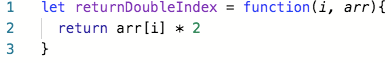Function that returns an index of an array doubled in O(1) time complexity.

For space, no data structures can be created that are a multiples of the size of n. Variables can be declared, but the number must not change with n.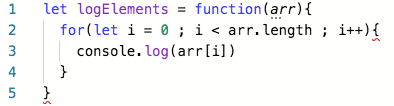Function that logs every element in an array with O(1) space.

# O(logn)

O(logn) is known as logarithmic complexity. The logarithm in O(logn) has a base of 2. The best way to wrap your head around this is to remember the concept of halving: every time n increases by an amount k, the time or space increases by k/2. There are several common algorithms that are O(logn) a vast majority of the time to keep an eye out for: binary search, searching for a term in a binary search tree, and adding items to a heap.

#algorithms #computer-science #big-o-notation #time-complexity #space-complexity #algorithms1598508720

## What Is Big O Notation?

As programmers, we often find ourselves asking the same two questions over and over again:

1. How much time does this algorithm need to complete?
2. How much space does this algorithm need for computing?

To put it in other words, in computer programming, there are often multiple ways to solve a problem, so

1. How do we know which solution is the right one?
2. How do we compare one algorithm against another?

The big picture is that we are trying to compare how quickly the runtime of algorithms grows with respect to the size of their input. We think of the runtime of an algorithm as a function of the size of the input, where the output is how much work is required to run the algorithm.

To answer those questions, we come up with a concept called Big O notation.

• Big O describes how the time is taken, or memory is used, by a program scales with the amount of data it has to work on
• Big O notation gives us an upper bound of the complexity in the worst case, helping us to quantify performance as the input size becomes arbitrarily large
• In short, Big O notation helps us to measure the scalability of our code

### Time and Space Complexity

When talking about Big O Notation it’s important that we understand the concepts of time and space complexity, mainly because_ Big O Notation_ is a way to indicate complexities.

Complexity is an approximate measurement of how efficient (or how fast) an algorithm is and it’s associated with every algorithm we develop. This is something all developers have to be aware of. There are 2 kinds of complexities: time complexity and space complexity. Time and space complexities are approximations of how much time and space an algorithm will take to process certain inputs respectively.

Typically, there are three tiers to solve for (best case scenario, average-case scenario, and worst-case scenario) which are known as asymptotic notations. These notations allow us to answer questions such as: Does the algorithm suddenly become incredibly slow when the input size grows? Does it mostly maintain its fast run time performance as the input size increases?

#performance #development #big o complexity #big o notation #big data1594945560

## Big O Notation and Time Complexity

Big O notation is a simplified analysis of an algorithm’s efficiency. Big O notation gives us an algorithm’s complexity in terms of input size, N. It gives us a way to abstract the efficiency of our algorithm or code from the machines/computers they run on. We don’t care how powerful our machine is, but rather, the basic steps of the code. We can use big O to analyze both time and space. I will go over how we can use Big O to measure time complexity using Ruby for examples.

Types of measurement

There are a couple of ways to look at an algorithm’s efficiency. We can examine worst-case, best-case, and average-case. When we examine big O notation, we typically look at the worst-case. This isn’t to say the other cases aren’t as important.

General rules

1. Ignore constants

5n ->O(n)

Big O notation ignores constants. For example, if you have a function that has a running time of 5n, we say that this function runs on the order of the big O of N. This is because as N gets large, the 5 no longer matters.

2. In the same way that N grows, certain terms “dominate” others

Here’s a list:

O(1) < O(logn) < O(n) < O(nlogn) < O(n²) < O(2^n) < O(n!)

We ignore low-order terms when they are dominated by high order terms.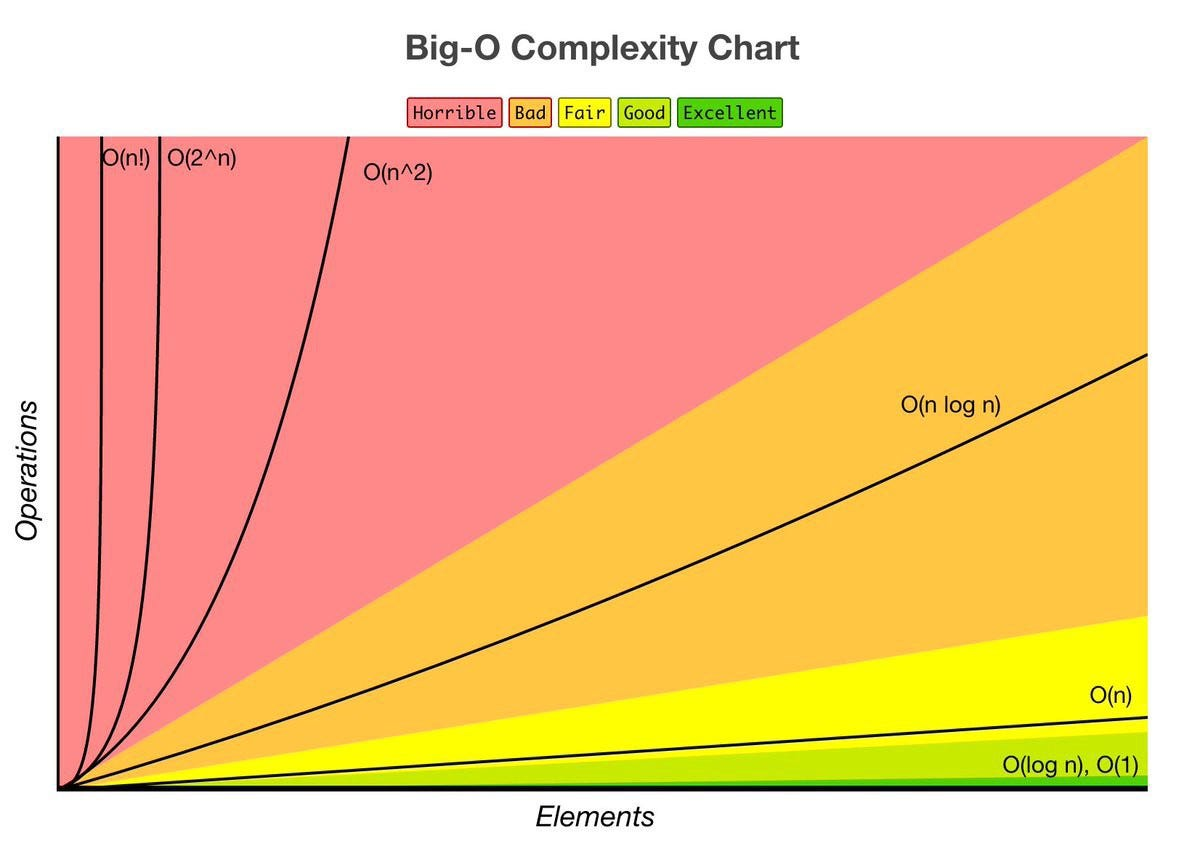Constant Time: O(1)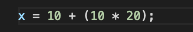This basic statement computes x and does not depend on the input size in any way. This is independent of input size, N. We say this is a “Big O of one” or constant time.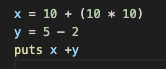total time = O(1) + O(1) + O(1) = O(1)

What happens when we have a sequence of statements? Notice that all of these are constant time. How do we compute big O for this block of code? We simply add each of their times and we get 3 * O(1). But remember we drop constants, so this is still big O of one.

Linear Time: O(n)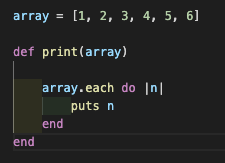The run time of this method is linear or O(n). We have a loop inside of this method which iterates over the array and outputs the element. The number of operations this loop performs will change depending on the size of the array. For example, an array of size 6 will only take 6 iterations, while an array of 18 elements would take 3 times as long. As the input size increases, so will the runtime.Big O notation is a simplified analysis of an algorithm’s efficiency. Big O notation gives us an algorithm’s complexity in terms of input size, N. It gives us a way to abstract the efficiency of our algorithm or code from the machines/computers they run on. We don’t care how powerful our machine is, but rather, the basic steps of the code. We can use big O to analyze both time and space. I will go over how we can use Big O to measure time complexity using Ruby for examples.

Types of measurement

There are a couple of ways to look at an algorithm’s efficiency. We can examine worst-case, best-case, and average-case. When we examine big O notation, we typically look at the worst-case. This isn’t to say the other cases aren’t as important.

General rules

#time-complexity #algorithms #big-o-notation #flatiron-school #algorithms1594945860

## Big O Notation and Time Complexity

Big O notation is a simplified analysis of an algorithm’s efficiency. Big O notation gives us an algorithm’s complexity in terms of input size, N. It gives us a way to abstract the efficiency of our algorithm or code from the machines/computers they run on. We don’t care how powerful our machine is, but rather, the basic steps of the code. We can use big O to analyze both time and space. I will go over how we can use Big O to measure time complexity using Ruby for examples.

Types of measurement

There are a couple of ways to look at an algorithm’s efficiency. We can examine worst-case, best-case, and average-case. When we examine big O notation, we typically look at the worst-case. This isn’t to say the other cases aren’t as important.

General rules

1. Ignore constants

5n ->O(n)

Big O notation ignores constants. For example, if you have a function that has a running time of 5n, we say that this function runs on the order of the big O of N. This is because as N gets large, the 5 no longer matters.

2. In the same way that N grows, certain terms “dominate” others

Here’s a list:

O(1) < O(logn) < O(n) < O(nlogn) < O(n²) < O(2^n) < O(n!)

We ignore low-order terms when they are dominated by high order terms.Constant Time: O(1)This basic statement computes x and does not depend on the input size in any way. This is independent of input size, N. We say this is a “Big O of one” or constant time.

#time-complexity #algorithms #big-o-notation #flatiron-school #algorithms